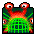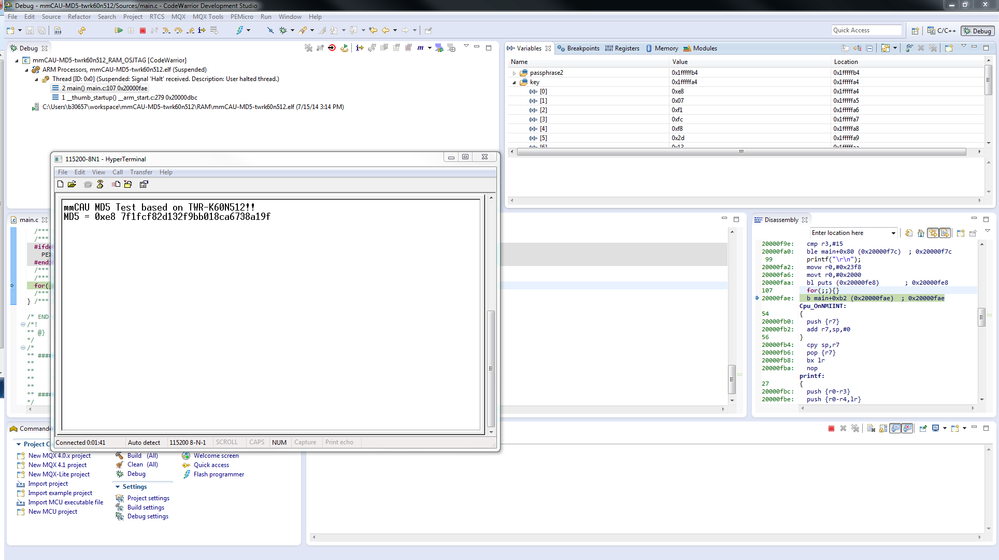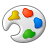# MD5 of MMCAU in K60

cancel
Showing results for
Show  only  | Search instead for
Did you mean:
SOLVED

## MD5 of MMCAU in K60

1,099 ViewsContributor III

Hi folks,

I tried to use MD5 with MMCAU library for K60 in MQX 3.8. Result: the hashes are not the same as we can calculate with our C# application and online calculators like MD5 - Online generator md5 hash

Our K60 has mask set 4N30D (Version1).

My questions:

Why did I got no valid hashes?

Does anybody use MD5 of MMCAU?

Are there any hints or tips you have?

our code fragments:

unsigned char passphrase2= "1234567890"; // there may by a 64 byte string

unsigned char key;

cau_md5_initialize_output(key);

cau_md5_hash_n(passphrase, 1, key);

// now key should be the md5 hash, but it is'nt

Caesar

Labels (1)
• ### Kinetis K Series MCUs

1 Solution
544 ViewsNXP TechSupport

Hi Caesarl,

Per MD5 spec, there is a pad process before feeding the raw message, please kindly refer to the following for details:

MD5 processes a variable-length message into a fixed-length output of 128 bits. The input message is broken up into chunks of 512-bit blocks (sixteen 32-bit words); the message is padded so that its length is divisible by 512. The padding works as follows: first a single bit, 1, is appended to the end of the message. This is followed by as many zeros as are required to bring the length of the message up to 64 bits fewer than a multiple of 512. The remaining bits are filled up with 64 bits representing the length of the original message, modulo 264.

Here I provide a pad() for that purpose, you may use it in your application, and please also note, if you use mmcau_md5_update() instead, no need to call cau_md5_initialize_output() firstly.

void pad(char *p, int num_blks, int nbytes)

{

int i,j,n;

for (i=0; i<(64*num_blks); i++) {

if (p[i] == 0)

break;

}

p[i++] = 0x80;

for (j=i; j<((64*num_blks)-8); j++)  {

p[j] = 0;

}

n = nbytes << 3;

p[j++] = n;

p[j++] = n>>8;

p[j++] = n>>16;

p[j++] = n>>24;

p[j++] = 0;

p[j++] = 0;

p[j++] = 0;

p[j] = 0;

}

I also attached my test project here, you may refer to it for more details.Hope that helps,

Have a great day!

B.R

Kan !

3 Replies
545 ViewsNXP TechSupport

Hi Caesarl,

Per MD5 spec, there is a pad process before feeding the raw message, please kindly refer to the following for details:

MD5 processes a variable-length message into a fixed-length output of 128 bits. The input message is broken up into chunks of 512-bit blocks (sixteen 32-bit words); the message is padded so that its length is divisible by 512. The padding works as follows: first a single bit, 1, is appended to the end of the message. This is followed by as many zeros as are required to bring the length of the message up to 64 bits fewer than a multiple of 512. The remaining bits are filled up with 64 bits representing the length of the original message, modulo 264.

Here I provide a pad() for that purpose, you may use it in your application, and please also note, if you use mmcau_md5_update() instead, no need to call cau_md5_initialize_output() firstly.

void pad(char *p, int num_blks, int nbytes)

{

int i,j,n;

for (i=0; i<(64*num_blks); i++) {

if (p[i] == 0)

break;

}

p[i++] = 0x80;

for (j=i; j<((64*num_blks)-8); j++)  {

p[j] = 0;

}

n = nbytes << 3;

p[j++] = n;

p[j++] = n>>8;

p[j++] = n>>16;

p[j++] = n>>24;

p[j++] = 0;

p[j++] = 0;

p[j++] = 0;

p[j] = 0;

}

I also attached my test project here, you may refer to it for more details.Hope that helps,

Have a great day!

B.R

Kan !

544 ViewsContributor III

Hello everybody!

Thanks for the discussion, thank you Kan for the explanation and the code.

It works perfectly for a short ASCII string.

I modified the pad function to work with binary (i.e. non-ASCII) data

and used cau_md5_upcate instead of cau_md5_hash.

I enclose the file md5_utils.c, which currently holds only the function pad().

(unfortunately copy&paste doesn't work here, otherwise I would just have pasted the code here)

As far as I have tested, the resulting hash matches the one obtained from an online md5 calculator.

Greetings,

Martin

544 ViewsContributor III

Hi Kan,

yeah! That's the hint I was looking for....

Now I can use MD5 and I'm happy.

Caesar